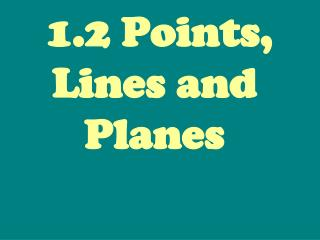DownloadDownload Presentation1.2 Points, Lines and Planes

# 1.2 Points, Lines and Planes

Download Presentation## 1.2 Points, Lines and Planes

- - - - - - - - - - - - - - - - - - - - - - - - - - - E N D - - - - - - - - - - - - - - - - - - - - - - - - - - -
##### Presentation Transcript

1. 1.2 Points, Lines and Planes

2. undefined terms There are three __________ ____________ in geometry. The following are not definitions, they are descriptions.

3. point • 1 A ______________ is the simplest figure studied in geometry. It doesn’t have any size. It is represented by a dot.

4. A C B All geometric figures consist of points. Below are points A, B and C. We name points with capital letters.

5. line • 2 A ________ extends in two directions without ending. It has no thickness.

6. l A B A line is referred to by a single lower case letter (line l ) or using two points on the line (AB or BA).

7. plane • 3 A __________ is suggested by a floor, wall or table top. However, it exists without ending and has no thickness.

8. We use a parallelogram to represent a plane. We label a plane with a capital letter. M N Plane M and Plane N.

9. We use the three undefined terms to define other concepts.

10. Collinear points • _________ _________ are points that lie on the same line. A C B D Points A, B, C are collinear. Points A, B and D are noncollinear.

11. Coplanar points • ___________ _________ points are points that lie on the same plane. • M, N, P, R are coplanar. • M, N, P, Q are noncoplanar. P N R M Q

12. The ____________of two figures is the set of points that are in both figures. intersection

13. P t Lines m and t intersect at point W. m W Point P is on line t.

14. M P k • k and P are in M. • M contains k and P • j intersects M at P. • P is the intersection of j and M. j

15. N X M Y Planes M and N intesect in line XY.

16. Ex 1. Using the figure given, list three sets of collinear points. • Ex 2. List a set of noncollinear points. A A, B, C B C E F, E, B F A, E, G D G A, B, D or F, E, C or A, E, C

17. X M T S O W R Y Ex 3. Copy this figure into your notebooks and then answer the T/F questions.

18. T F • A) Line XY intersects M at point O. • B) Plane M intersects line XY in more than one point. • C) T, O, and R are collinear. • D) X, O, and Y are collinear. • E) R, O, S, and W are coplanar. • F) R, S, T, and X are coplanar. • G) R, X, O and Y are coplanar. F T T F T

19. Ex 4. Name a fourth point that is in the same plane as the given points. • a) A, B, C • b) D, C, H • c) B, E, F • d) D, B, F D G A D C H A B H G E F • The plane that contains the top of the box • can be called plane ABCD. (Go around in order)

20. Ex 5. • Are there more than 4 points in plane ABCD? • Are there any points in line CG besides C and G? • Name the intersection of planes ABFE and BCGF. • Name two planes that do not intersect. Yes!! Yes!! ABCD and EFGH ADHE and BCGF ABFE and DCGH

21. Segment AC ____________________ consists of points A and C and all points that are between A and C. • A and C are called the _____________ of segment AC. A C endpoints

22. __________________ consists of the initial point A and all the points on line AC that lie on the same side of A as point C. Ray AC A C Name the endpoint first.

23. S T R Ex 6. Write 3 times when the hands of the clock are opposite rays. 3:45 12:30 9:15 6:00